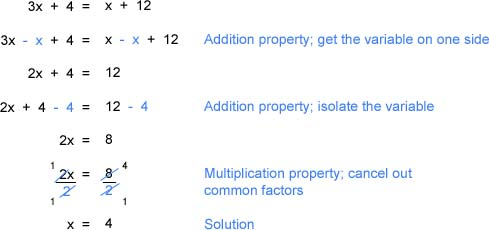Linear Equations

Linear Equations

The overriding principle in solving linear equations is to isolate the variable.

At times, the variable expressions or other like terms may need to be combined first.   Then the variable can be isolated by performing inverse operations to both sides of the equation.

When solving linear equations, clearly document each step in the process so that you can avoid mistakes or catch errors when checking your work.

Example

Solve:     13 - (2x + 2) = 2(x + 2) + 3x

Multiply across the parentheses using the distributive property.  If there are minus signs in front of parentheses, they get distributed as well:

13 - (2x + 2) = 2(x + 2) + 3x

13 - 2x - 2 = 2x + 4 + 3x

Combine like terms:

13 - 2x - 2 = 2x + 4 + 3x

11 - 2x = 5x + 4

Get the variables on one side of the equation, and the constants (terms without variables) on the other side by performing inverse operations on both sides:

11 - 2x + 2x = 5x + 4 + 2x

11 = 7x + 4

11 - 4 = 7x + 4 - 4

7 = 7x

Perform one more inverse operation (either multiplication or division) to get the variable by itself:

7/ 7 = 7x/ 7

1 = x

Example 2 - Solve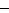2x5 =3

First add 5 to both sides2x5 + 53 + 5

Then multiply both sides by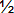2x(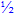) = 2()

x1

Example 3 - Solve for the following expression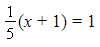Multiply both sides by the LCD, 5.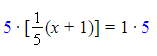x + 1 = 5

x = 4

Example 4